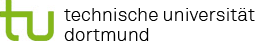You are here:

### Project P2: Model selection for exposure time-dose curves with mixture models

In project P2, mixture models of parametric and nonparametric functions for modelling dose-expression curves will be developed.

Parametric models, often based on logistic functions, are increasingly used to model doseresponse curves. Since the parametric form is usually not known a priori, often model selection between different given parametric models is performed, taking into account the additional statistical uncertainty of the model choice. MCP-Mod ("Multiple Comparison Procedures Modelling"), which is particularly popular in the pharmaceutical industry, is a statistical procedure that enables a structured combination between hypothesis testing and such a curve modelling approach with model selection (Bretz et al., 2005).

Parametric curves can provide biased estimates when the model assumptions are not fulfilled. For verification purposes, deviations from sigmoid curves, for example, can be measured. Alternatively, dose-response curves can be adjusted using nonparametric methods, such as kernel regression (Staniswalis and Cooper, 1988) or local linear regression (Zhang et al., 2013). These methods can also capture structures that a misspecified parametric model cannot capture. However, they often lead to estimates with high variance. As a compromise, a semiparametric approach using a mixture model can be used. For example, Yuan and Yin (2011) suggest a weighted average of estimates of parametric and non-parametric adjustments as estimators, where the weights depend on the quality of the respective adjustments.

The first step in this project will be to investigate which of these methods are particularly suitable for expression-response curves. In preliminary studies, as part of a master thesis in which MCP-Mod was applied to genome-wide expression data, we found that for different genes different parametric models lead to the best fits.

The IfADo generates data in which not only the concentration of a compound but also the exposure time is varied (Gu et al., 2018). In this project, the methods from one-dimensional modelling are transferred to the two-dimensional situation, when exposure time-dose curves must be estimated. This is a new approach, and there is much flexibility for the type of modelling. In a first approach, the established methods for dose-response curves will be used separately for each exposure time, and then the results can be compared over the different exposure times and possibly combined. Further, new models for two-dimensional exposure time-dose expression curves will be developed. Direct extensions of the one-dimensional models are promising. Since multiple testing in the first step of MCP-Mod can also be extended to the two-dimensional case, a two-dimensional variant of MCP-Mod will be developed. Another idea is to model a coupling between the curves for different genes. For the calculation of differential gene expression between two groups of experiments, this principal approach has led to the extremely popular Limma method, in which gene specific variances are jointly modelled in an empirical Bayes approach (Smyth, 2004; Smyth, 2005). In this project, parameters of the modelled curves will be estimated accordingly, leading to regularisation of the parameters towards mean.

#### References

• Bretz J, Pinheiro CP, Branson M (2005). Combining multiple comparisons and modelling techniques in dose-response studies. Biometrics 61(3), 738-48, doi: 10.1111/j.15410420.2005.00344.x.
• Gu X, Albrecht W, Edlund K, Kappenberg F, Rahnenführer J, Leist M, et al. (2018). Relevance of the incubation period in cytotoxicity testing with primary human hepatocytes. Archives of Toxicology 92(12), 3505–3515, doi:10.1007/s00204-018-2302-0.
• Smyth GK (2004). Linear models and empirical Bayes methods for assessing differential expression in microarray experiments. Statistical Applications in Genetics and Molecular Biology 3(3), 1-25, doi: 10.2202/1544-6115.1027.
• Smyth GK (2005). Limma: linear models for microarray data. Bioinformatics and computational biology solutions using R and Bioconductor. Springer, New York, NY, 397-420, doi: 10.1007/0387-29362-0_23.
• Staniswalis J, Cooper V (1988). Kernel estimates of dose response. Biometrics 44, 1103–1119, doi: 10.2307/2531739.
• Yuan Y, Yin G (2011). Dose-response curve estimation: A semiparametric mixture approach. Biometrics 67, 1543–1554, doi: 10.1111/j.1541-0420.2011.01620.x.
• Zhang H, Holden-Wiltse J, Wang J, Liang H (2013). A strategy to model nonmonotonic doseresponse curve and estimate IC50. PLOS ONE 8 (8), doi: 10.1371/journal.pone.0069301.